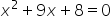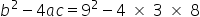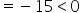Mathematics
Easy

Question

# How many solutions are there for the equation 3using discriminant?

## 0    1    2    Either 1 or 2.Hint:

## The correct answer is: 0

### Step 1 of 1:We have given an equation 3Now,The discriminant value is less than zero, so it has no real solution.

The discriminant is the part of the quadratic formula found within the square root.

### Related Questions to study#### With Turito Foundation.#### Get an Expert Advice From Turito.